Next: Assessing as a feature Up: Feature selection Previous: Mutual information   Contents   Index

##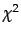Feature selectionChi2 Feature selection

Another popular feature selection method is. In statistics, thetest is applied to test the independence of two events, where two events A and B are defined to be independent if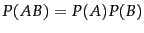or, equivalently,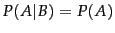and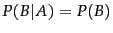. In feature selection, the two events are occurrence of the term and occurrence of the class. We then rank terms with respect to the following quantity: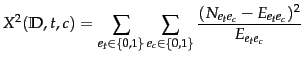(133)

where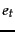and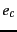are defined as in Equation 130.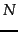is the observed frequency in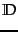and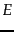the expected frequency. For example,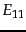is the expected frequency of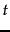and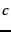occurring together in a document assuming that term and class are independent.

Worked example. We first computefor the data in Example 13.5.1: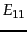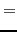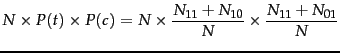(134)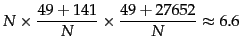(135)

where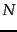is the total number of documents as before.

We compute the other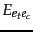in the same way: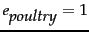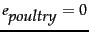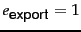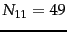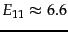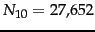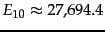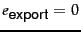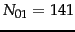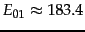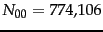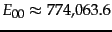Plugging these values into Equation 133, we get a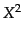value of 284: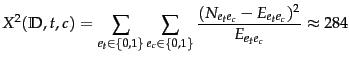(136)

End worked example.is a measure of how much expected countsand observed countsdeviate from each other. A high value ofindicates that the hypothesis of independence, which implies that expected and observed counts are similar, is incorrect. In our example,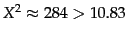. Based on Table 13.6 , we can reject the hypothesis that poultry and export are independent with only a 0.001 chance of being wrong.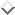Equivalently, we say that the outcome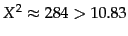is statistically significant at the 0.001 level. If the two events are dependent, then the occurrence of the term makes the occurrence of the class more likely (or less likely), so it should be helpful as a feature. This is the rationale offeature selection.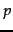critical value 0.1 2.71 0.05 3.84 0.01 6.63 0.005 7.88 0.001 10.83

An arithmetically simpler way of computing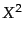is the following: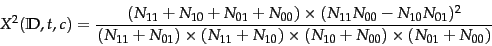(137)

This is equivalent to Equation 133 (Exercise 13.6 ).

SubsectionsNext: Assessing as a feature Up: Feature selection Previous: Mutual information   Contents   Index